# Java Web—【分页功能】详解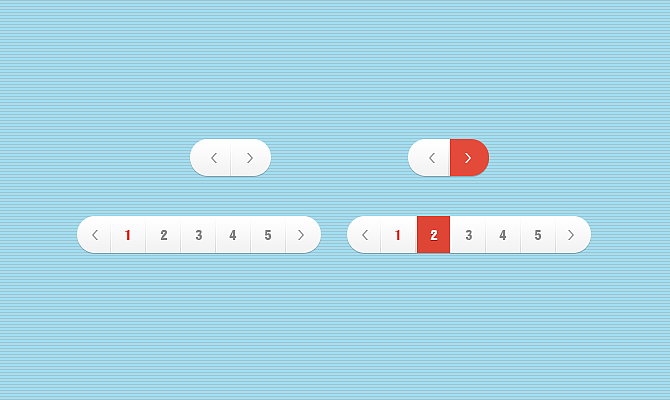## 分页简介

• 使用场景： 当取到的数据量达到一定的时候，就需要使用分页来进行数据分割。

• 客户端的问题： 如果数据量太多，都显示在同一个页面的话，会因为页面太长严重影响到用户的体验，也不便于操作，也会出现加载太慢的问题。
• 服务端的问题： 如果数据量太多，可能会造成内存溢出，而且一次请求携带的数据太多，对服务器的性能也是一个考验。

#### 分页的分类

1.真分页（物理分页）：

• 实现原理： SELECT * FROM xxx [WHERE...] LIMIT #{param1}, #{param2}
第一个参数是开始数据的索引位置
第二个参数是要查询多少条数据
• 优点： 不会造成内存溢出
• 缺点： 翻页的速度比较慢

2.假分页（逻辑分页）：

• 实现原理： 一次性将所有的数据查询出来放在内存之中，每次需要查询的时候就直接从内存之中去取出相应索引区间的数据
• 优点： 分页的速度比较快
• 缺点： 可能造成内存溢出

## 传统的分页方式

#### 分页的原理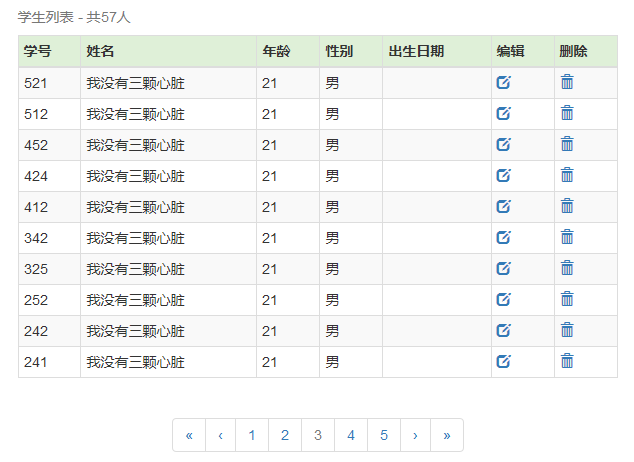• 页面中的数据有：
结果集：通过 SQL 语句查询得来的——List
• 分页条中的数据有：
当前页：用户传递到后台——currentPage
总页数：计算的来——totalPage
上一页：计算的来——prePage
下一页：计算的来——nextPage
尾页：计算的来（总页数）——lastPage
页面大小（即每一页显示的条数）：用户传递到后台——count
总条数：通过 SQL 语句查询得来的——totalCount

• SELECT * FROM student LIMIT #{param1}, #{param2}
• SELECT COUNT(*) FROM student

• 总页数：totalPage
总页数 = 总条数 % 页面大小 == 0 ? 总条数 / 页面大小 : 总条数 / 页面大小 + 1
• 上一页：prePage
上一页 = 当前页 - 1 > = 1 ? 当前页 - 1 : 1
• 下一页：nextPage
下一页 = 当前页 + 1 <= totalPage ? 当前页 + 1 : totalPage
• 尾页：lastPage
尾页 = 总条数 % 页面大小 == 0 ？ 总条数 - 页面大小 : 总条数 - 总条数 % 页面大小

• 当前页：currentPage
• 页面大小：count

public class Page {

int start;        // 开始数据的索引
int count;        // 每一页的数量
int total;        // 总共的数据量

/**
* 提供一个构造方法
* @param start
* @param count
*/
public Page(int start, int count) {
super();
this.start = start;
this.count = count;
}

/**
* 判断是否有上一页
* @return
*/
public boolean isHasPreviouse(){
if(start==0)
return false;
return true;

}

/**
* 判断是否有下一页
* @return
*/
public boolean isHasNext(){
if(start==getLast())
return false;
return true;
}

/**
* 计算得到总页数
* @return
*/
public int getTotalPage(){
int totalPage;
// 假设总数是50，是能够被5整除的，那么就有10页
if (0 == total % count)
totalPage = total /count;
// 假设总数是51，不能够被5整除的，那么就有11页
else
totalPage = total / count + 1;

if(0==totalPage)
totalPage = 1;
}

/**
* 计算得到尾页
* @return
*/
public int getLast(){
int last;
// 假设总数是50，是能够被5整除的，那么最后一页的开始就是45
if (0 == total % count)
last = total - count;
// 假设总数是51，不能够被5整除的，那么最后一页的开始就是50
else
last = total - total % count;

last = last<0?0:last;
return last;
}

/* getter and setter */
}

## 前台实现分页设计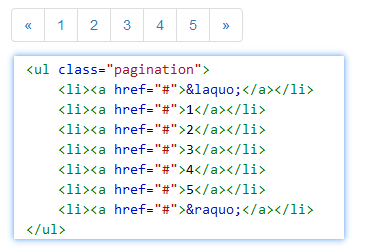#### 简单版本的分页条

• 首页超链：指向了 start 为 0 的首页
<li>
<a href="?page.start=0">
<span>«</span>
</a>
</li>
• 上一页超链：
<li >
<a  href="?page.start=${page.start-page.count}"> <span>‹</span> </a> </li> • 下一页超链： <li > <a href="?page.start=${page.start+page.count}">
<span>›</span>
</a>
</li>
• 最后一页超链：指向了最后一页
<li >
<a href="?page.start=${page.last}"> <span>»</span> </a> </li> • 中间页： <c:forEach begin="0" end="${page.totalPage-1}" varStatus="status">
<li>
<a href="?page.start=${status.index*page.count}" class="current">${status.count}</a>
</li>
</c:forEach>
• 所以写完看起来会是这样子的：
<nav>
<ul class="pagination">
<li>
<a  href="?page.start=0">
<span>«</span>
</a>
</li>

<li >
<a  href="?page.start=${page.start-page.count}"> <span>‹</span> </a> </li> <c:forEach begin="0" end="${page.totalPage-1}" varStatus="status">
<li>
<a href="?page.start=${status.index*page.count}" class="current">${status.count}</a>
</li>
</c:forEach>

<li >
<a href="?page.start=${page.start+page.count}"> <span>›</span> </a> </li> <li > <a href="?page.start=${page.last}">
<span>»</span>
</a>
</li>
</ul>
</nav>
• 存在的问题：
① 没有边界判断，即在首页仍然可以点击前一页，不符合逻辑也影响用户体验
② 会显示完所有的分页，即如果 totalPage 有50页，那么分页栏将会显得特别长，影响体验

#### 改良版本的分页条

1.写好头和尾

<nav class="pageDIV">
<ul class="pagination">
.....
</ul>
</nav>

2.写好« ‹这两个功能按钮

        <li <c:if test="${!page.hasPreviouse}">class="disabled"</c:if>> <a href="?page.start=0"> <span>«</span> </a> </li> <li <c:if test="${!page.hasPreviouse}">class="disabled"</c:if>>
<a href="?page.start=${page.start-page.count}"> <span>‹</span> </a> </li> 再通过 JavaScrip 代码来完成禁用功能： <script>$(function () {
$("ul.pagination li.disabled a").click(function () { return false; }); }); </script> 3.完成中间页码的编写 <c:forEach begin="0" end="${page.totalPage-1}" varStatus="status">

<c:if test="${status.count*page.count-page.start<=30 && status.count*page.count-page.start>=-10}"> <li <c:if test="${status.index*page.count==page.start}">class="disabled"</c:if>>
<a
href="?page.start=${status.index*page.count}" <c:if test="${status.index*page.count==page.start}">class="current"</c:if>
>\${status.count}</a>
</li>
</c:if>
</c:forEach>

0 循环到 page.totalPage - 1varStatus 相当于是循环变量

• status.count 是从1开始遍历
• status.index 是从0开始遍历
• 要求：显示当前页码的前两个和后两个就可，例如当前页码为3的时候，就显示 1 2 3(当前页) 4 5 的页码
• 理解测试条件：
• 10 <= 当前页*每一页显示的数目 - 当前页开始的数据编号 <= 30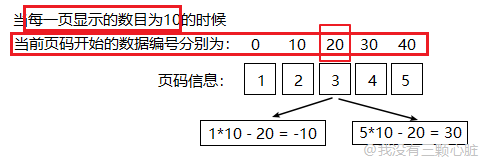• 只要理解了这个判断条件，其他的就都好理解了
• 注意： 测试条件是需要根据项目的需求动态改变的，不是万能的！

## 后台中的分页

public List<Student> list() {
return list(0, Short.MAX_VALUE);
}

public List<Student> list(int start, int count) {

List<Student> students = new ArrayList<>();

String sql = "SELECT * FROM student ORDER BY student_id desc limit ?,?";

try (Connection c = DBUtil.getConnection(); PreparedStatement ps = c.prepareStatement(sql)) {

ps.setInt(1, start);
ps.setInt(2, count);

// 获取结果集...
} catch (SQLException e) {
e.printStackTrace();
}
return students;
}

// 获取分页参数
int start = 0;
int count = 10;

try {
start = Integer.parseInt(req.getParameter("page.start"));
count = Integer.parseInt(req.getParameter("page.count"));
} catch (Exception e) {
}
Page page = new Page(start, count);

List<Student> students = studentDAO.list(page.getStart(), page.getCount());

....

// 共享数据
req.setAttribute("page", page);
req.setAttribute("students", students);

## SSM 中的分页

#### 第一步：添加相关 jar 依赖包

PageHelper 需要依赖两个 jar 包，我们直接在 pom.xml 中增加两个 jar 包依赖：

<!-- pageHelper -->
<dependency>
<groupId>com.github.pagehelper</groupId>
<artifactId>pagehelper</artifactId>
<version>5.1.2-beta</version>
</dependency>

<!--jsqlparser-->
<dependency>
<groupId>com.github.jsqlparser</groupId>
<artifactId>jsqlparser</artifactId>
<version>1.0</version>
</dependency>

#### 第二步：配置相关环境

<!-- 配置SqlSessionFactory对象 -->
<bean id="sqlSessionFactory" class="org.mybatis.spring.SqlSessionFactoryBean">
<!-- 注入数据库连接池 -->
<property name="dataSource" ref="dataSource"/>
<!-- 扫描entity包 使用别名 -->
<property name="typeAliasesPackage" value="cn.wmyskxz.entity"/>
<!-- 扫描sql配置文件:mapper需要的xml文件 -->
<property name="mapperLocations" value="classpath:mapper/*.xml"/>
<!-- 让MyBatis支持PageHelper插件 -->
<property name="plugins">
<array>
<bean class="com.github.pagehelper.PageInterceptor">
<property name="properties">
<!--使用下面的方式配置参数，一行配置一个 -->
<value>
</value>
</property>
</bean>
</array>
</property>
</bean>

#### 第三步：重构项目

<!-- 查询从start位置开始的count条数据-->
<select id="list" resultMap="student">
SELECT * FROM student ORDER BY student_id desc
</select>

<!--&lt;!&ndash; 查询数据条目 &ndash;&gt;-->
<!--<select id="getTotal" resultType="int">-->
<!--SELECT COUNT(*) FROM student-->
<!--</select>-->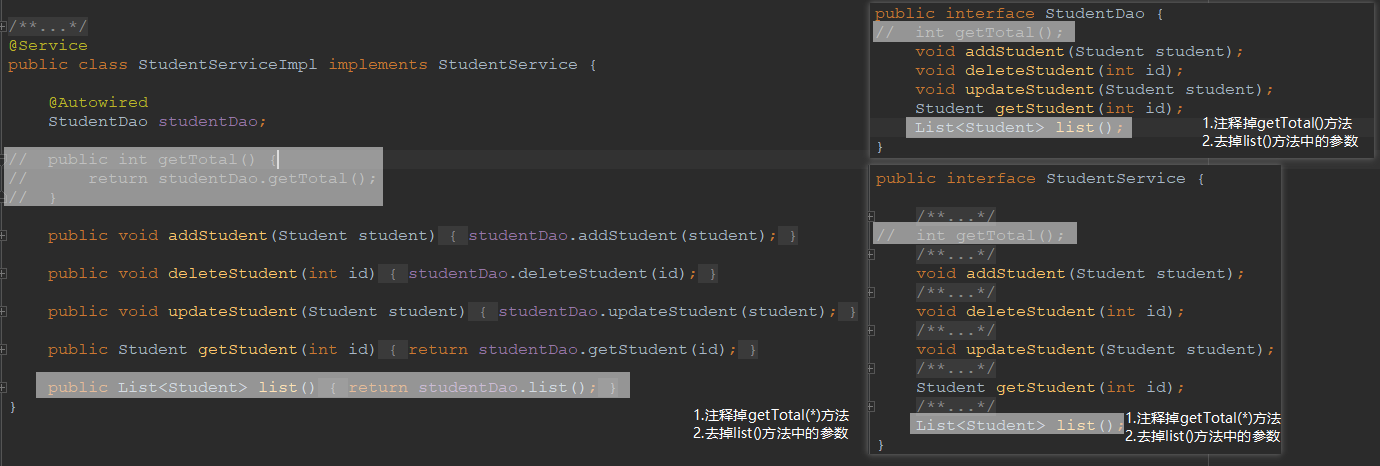@RequestMapping("/listStudent")
public String listStudent(HttpServletRequest request, HttpServletResponse response) {

// 获取分页参数
int start = 0;
int count = 10;

try {
start = Integer.parseInt(request.getParameter("page.start"));
count = Integer.parseInt(request.getParameter("page.count"));
} catch (Exception e) {
}

Page page = new Page(start, count);

//  使用 PageHelper 来设置分页
PageHelper.offsetPage(page.getStart(),page.getCount());
List<Student> students = studentService.list();
//  使用 PageHelper 来获取总数
int total = (int) new PageInfo<>(students).getTotal();
page.setTotal(total);

request.setAttribute("students", students);
request.setAttribute("page", page);

return "listStudent";
}

github：wmyskxz